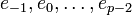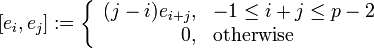# Characteristic Lie subring not implies ideal

ANALOGY BREAKDOWN: This is the breakdown of the analogue in Lie rings of a fact encountered in group. The old fact is: characteristic implies normal.
View other analogue breakdowns of characteristic implies normal|View other analogue breakdowns from group to Lie ring
This article gives the statement and possibly, proof, of a non-implication relation between two Lie subring properties. That is, it states that every Lie subring satisfying the first Lie subring property (i.e., characteristic Lie subring) need not satisfy the second Lie subring property (i.e., ideal of a Lie ring)
View a complete list of Lie subring property non-implications | View a complete list of Lie subring property implications
Get more facts about characteristic Lie subring|Get more facts about ideal of a Lie ring

## Statement

A characteristic subring of a Lie ring need not be an ideal of the Lie ring.

## Proof

### Example of the simple Witt algebra

Suppose$p$ is a prime number greater than 3. Let$\mathbb{F}_p$ be the prime field for the prime$p$. Denote by$L$ the simple Witt algebra for$\mathbb{F}_p$ corresponding to the prime$p$; explicitly, this means that:

• The additive group has basis$e_{-1},e_0,\dots,e_{p-2}$. Explicitly, it is$\bigoplus_{i=-1}^{p-2} Fe_i$.
• The Lie bracket is defined as follows on the basis:$[e_i,e_j] := \left \lbrace\begin{array}{rl} (j - i)e_{i+j}, & -1 \le i + j \le p - 2 \\0, & \mbox{otherwise}\\\end{array}\right.$

Consider the "sandwich" Lie subring$S$ of$L$ given by:$S = \bigoplus_{2i > p} \mathbb{F}_pe_i$

•$S$ is clearly a subring of$L$.
•$S$ is characteristic in$L$, because$x \in S \iff [x,[x,y]] = 0 \ \forall \ y \in L$ (note that the set with this description is not always a subring, but in this case it is).
•$S$ is not an ideal in$L$: for instance,$[S,e_{-1}]$ is not in$S$. In fact,$L$ is simple, so it has no proper nonzero ideal.